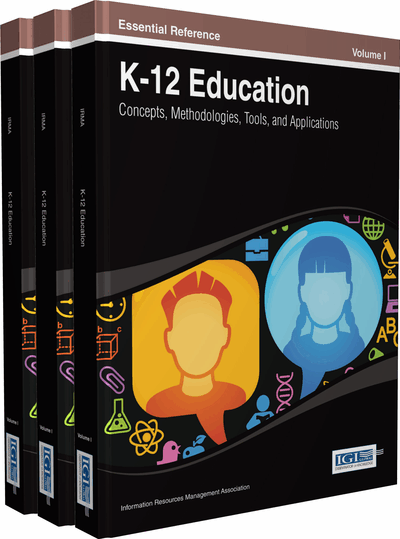# Do Technologies Support the Implementation of the Common Core State Standards in Mathematics of High School Probability and Statistics?

Woong Lim, Dong-Gook Kim
DOI: 10.4018/978-1-4666-4502-8.ch073
OnDemand:
(Individual Chapters)
Available
\$29.50
No Current Special Offers

## Abstract

This chapter reviews the roles of technology in statistics education and introduces technologies available for classroom use. A few concrete examples of how select technologies support the teaching of probability and statistics guided by the Common Core State Standards in Mathematics of high school Probability and Statistics (CCSSM-PS) are presented. The reality of the implementation of the CCSSM poses a rather exciting opportunity for all of us in mathematics education. It presents an opportunity to plan and create mathematics lessons based on good teaching strongly tied with technology. As the efficacy of the CCSSM-PS hinges on how teachers draw upon their content knowledge to facilitate student learning through technology, it is significant to provide professional development programs that help teachers infuse technology with the teaching of probability and statistics.
Chapter Preview
Top

## Introduction

The Common Core State Standards in Mathematics of high school Probability and Statistics (CCSSM-PS) present an overarching conception of statistics. The standards have domains organized sequentially so that students can develop an understanding of statistics as the subject area that provides tools for explaining variability in data that enables students to make informed decisions or predictions. The four domains are as follows:

• 1.

Interpreting data,

• 2.

Making inferences and justifying conclusions,

• 3.

Conditional probability, and

• 4.

Making probability-based decisions.

The CCSSM-PS go beyond addressing descriptive statistics and introduce key ideas for understanding inferential statistics. The standards introduce the conditional probability, the rules of probability, and using probabilities to make informed decisions.

Statistics is a powerful tool in scientific methods to turn data into a cohesive unit of findings, interpretations, and informed decisions. For example, based on the sample data, one computes numbers to represent the sample and uses the numbers to better grasp the larger group called population (i.e., parameters). One can also make predictions about the likelihood of an event of interest by computing a probability based on mathematical representations of the population (or sample) distributions. The CCSSM-PS introduce discrete probability distributions or probability density functions as such representation. The discussion of the probability density functions of the normal distribution, however, does not appear in the standards, presumably because the topic involves the use of calculus.

The CCSSM-PS are closely related to statistical thinking that highlights several key components including process thinking; understanding variation; and using data to guide decision-making (Snee, 1990). Statistical thinking acknowledges that all processes in a system of input and output exhibit variation. It manages uncertainty by quantifying the variation, and the language of uncertainty is probability. That is, through statistical thinking researchers examine a system of process consisting of input and output; perform data collection and analysis to identify variation to address uncertainty; and technology enhances the ability of practitioners of statistics to solve many problems in the analysis of data (Rubin & Hammerman, 2006). In this way, statistical thinking, a major philosophy of the CCSSM in framing statistical education, is related to learning to think critically facilitated with technology, and each domain in the CCSSM-PS is not left out as a stand-alone area but remain a part of interconnected body of knowledge.

A brief summary of the new directions in CCSSM-PS is as follows:

• Presenting a comprehensive view of statistics as a tool for data analysis and decision making.

• Promoting statistical thinking, which can be as essential as reading and writing for efficient citizenship (Wallman, 1993).

• Providing a sequential structure of key topics.

• Emphasizing building practical (rather than theoretical) knowledge of probability, such as calculating probabilities of compound events for inferential statistics.

• Proposing the probability distributions and the expected value of random variables as topics in order to be college and career ready.

This chapter aims to explain the role of technology in these new directions. In an effort to do so, this chapter demonstrates multiple roles of technology in statistics education and introduces technologies available for classroom use. A few concrete examples of how select technologies support the teaching of probability and statistics guided by CCSSM-PS are presented. The chapter demonstrates how good teaching can integrate technology in order to address specific standards and calls for more supports for designing and implementing effective teacher development programs that help teachers infuse technology with the teaching of probability and statistics.

## Complete Chapter List

Search this Book:
Reset